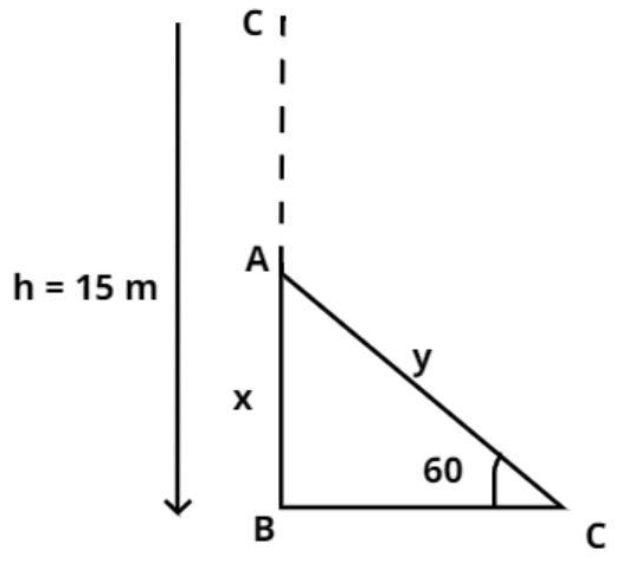QuestionAnswers

# A vertically straight tree, 15 m high, is broken by the wind in such a way that its top just touches the ground and makes an angle of 60 degrees with the ground. At what height from the ground did the tree break?Verified
155.1k+ views
Hint – In this question CB is the height of the tree and it is being broken from top after a certain point. Let A be the point after which it is broken, it is given that this broken part touches the ground and makes an angle of 60 degrees, so let AC be the broken part and use trigonometric ratios to find out the length below the point A for the tree.Let us consider the vertically straight tree BC as shown in the figure which is 15 m high.
Now the tree is broken by the wind that its top just touches the ground at C and makes an angle 60 degree from the ground.
Let us assume that the tree is broken at point A (see figure) and the point C touches the ground (see figure) and it is given that the point C makes an angle 60 degree from the ground (see figure).
So, we have to calculate the value of AB.
Let us assume BA be x meter and AC be y meter.
$\Rightarrow BC = BA + AC$
$\Rightarrow 15 = x + y$………………………….(1)
Now in triangle ABC
$\sin {60^0} = \dfrac{{{\text{Perpendicular}}}}{{{\text{Hypotenuse}}}} = \dfrac{x}{y}$
Now apply the value of $\sin {60^0} = \dfrac{{\sqrt 3 }}{2}$ in above equation we have,
$\Rightarrow \dfrac{{\sqrt 3 }}{2} = \dfrac{x}{y}$
From equation (1) $y = 15 - x$ so, substitute the value of y in above equation we have,
$\Rightarrow \dfrac{{\sqrt 3 }}{2} = \dfrac{x}{{15 - x}}$
Now simplify the above equation we have,
$\Rightarrow 15\sqrt 3 - x\sqrt 3 = 2x$
$\Rightarrow x\left( {2 + \sqrt 3 } \right) = 15\sqrt 3$
$\Rightarrow x = \dfrac{{15\sqrt 3 }}{{2 + \sqrt 3 }}{\text{ meter}}{\text{.}}$
So, this is the required height from the ground from which the tree is broken.

Note – Whenever we face such types of problems the key concept is to have a diagrammatic representation of the information provided in the question, this will help in understanding the basic outline of the triangle in which we need to apply trigonometric ratios. This concept will help you get on the right track to reach the answer.